# Chapter 6: Applications of Vector Algebra - Online Test

Q1. Ifand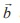are parallel vectors, then [] is equal to
Explaination / Solution:
No Explaination.

Q2. If a vector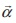lies in the plane of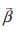and, then
Explaination / Solution:
No Explaination.

Q3. If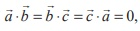, then the value of [] is
Explaination / Solution:
No Explaination.

Q4. Ifare three unit vectors such thatis perpendicular to, and is parallel tothenx (x) is equal to
Explaination / Solution:
No Explaination.

Q5. If [,] = 1, then the value of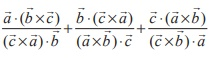is
Explaination / Solution:
No Explaination.

Q6. The volume of the parallelepiped with its edges represented by the vectors iˆ + ˆj, iˆ + 2 ˆj, iˆ + ˆj + π kˆ is
Explaination / Solution:
No Explaination.

Q7. Ifandare unit vectors such that [, b,× b ] = π/4, then the angle betweenandis
Explaination / Solution:
No Explaination.

Q8. If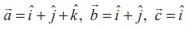and, then the value of λ + μ is
Explaination / Solution:
No Explaination.

Q9. Ifare non-coplanar, non-zero vectors such that [] = 3, thenis equal to
Explaination / Solution:
No Explaination.

Q10. Ifare three non-coplanar vectors such that, then the angle betweenandis
Explaination / Solution:
No Explaination.